## Tuesday, May 19, 2020

### Unizor - Physics4Teens - Electromagnetism - Magnetic Field - Lorentz Force

Notes to a video lecture on http://www.unizor.com

Magnetism - Lorentz Force

In this lecture we will look at the interaction between an electric current and a magnetic field.

We start with an analogy between magnetic properties of a wire loop with
electric current running through it and those of a permanent magnet.
This have been explained in the previous session from the position of Ampere model of magnetism.

The picture below illustrates this analogy.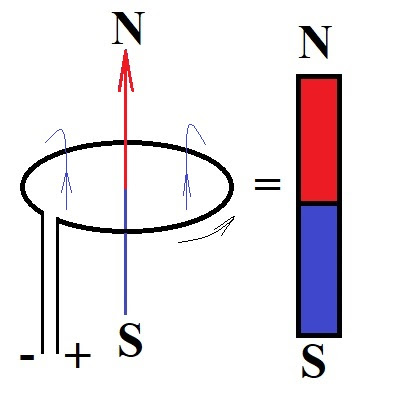The wire loop with electric current running through it (thin black arrow
from left to right) creates a magnetic field around it. The lines of
this magnetic field (thin dark blue arrows from bottom up) go through
the wire loop and around it, closing on themselves, forming their own
loops. Inside the wire loop the direction of magnetic lines is from
South pole to North, while outside the wire loop they go from North pole
to South. Those magnetic filed line loops that are on the same distance
from the wire make up a tubular surface (a torus) around the wire.

This wire loop with electric current running through it and a magnetic
field around it would behave like a magnet, like a compass arrow, for
example.

In particular, positioned inside some external magnetic field, like in
the magnetic field of the Earth, and allowed to turn free, it will
orient itself in such a way that its North pole will point to a South
pole of an external magnetic field, which, in case of the magnetic field
of the Earth, is located not far from its geographical North pole.

It should be noted that circular form of a wire loop is not essential.
If it's rectangular, the magnetic behavior will be the same. It is,
actually, more convenient to work with a rectangular frame to illustrate
the interaction of magnetic field and electric current.

Let's start the experiment with a rectangular wire loop, that can rotate
around a vertical axis in the external magnetic field. Position it such
that one vertical segment of a wire is close to one pole of an external
magnet, while an opposite side is close to another pole. Let the
electric current run through it.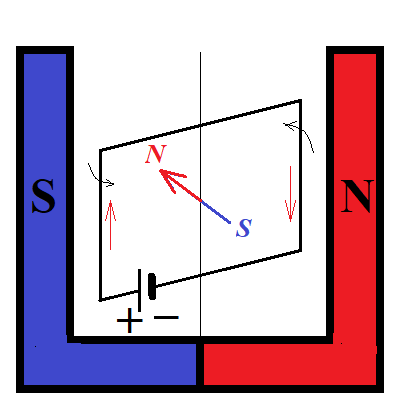If this wire frame with electric current running through it is allowed
to rotate around a vertical axis, it will reorient itself with its North
pole directed to the left towards the South pole of an external magnet,
and its South pole directed to the right towards the North pole of an
external magnet, as shown on a picture below. The distance from the
North pole of an external magnetic field to both vertical sides of a
wire will be the same. Same about the South pole of the external
magnetic field.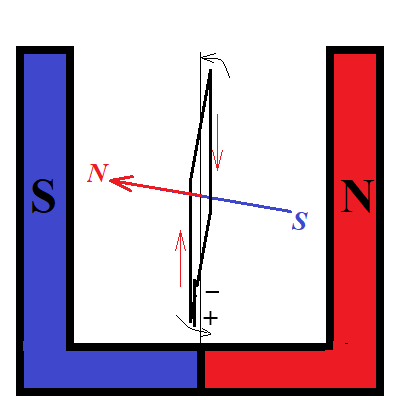This turn of a wire is, obviously, the result of forces of interaction
between external magnetic field and electric current with its own
magnetic field around it.

Consider the same two states of a wire (before and after the turn) viewed from above.

The initial position of a wire, viewed from above with rotating forces acting on it (blue arrows) is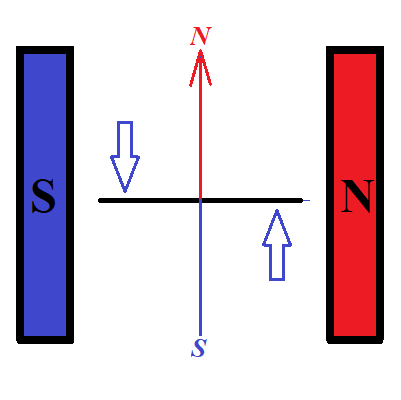Magnetic field lines of a wire with electric current running through it
are oriented along vertical direction on this picture, while the
magnetic field lines of an external magnetic field are horizontal.

As in the case of a compass arrow, aligning itself along the magnetic
field lines of the Earth, the external magnetic field forces, acting on
the magnetic field of a wire (blue arrows), will turn the wire to orient
its magnetic field lines along the magnetic field lines of an external
magnetic field, as shown on the following picture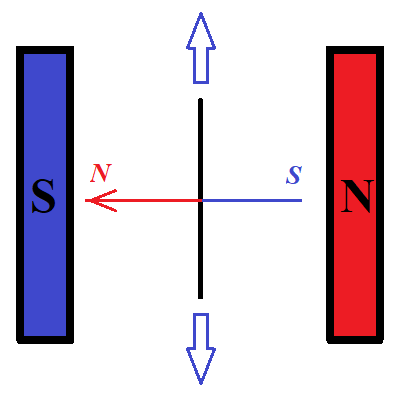At this final position external magnetic field forces (blue arrows) are
balancing each other and the rotation of a wire (after a short wobbling)
will stop.

Let's analyze the forces acting on a wire to turn it this way.

For this we don't really need a wire loop of any shape, it's sufficient
to have a linear wire with electric current running through it
positioned in an external magnetic field.

If we open up a wire loop into a straight line with electric current
running through it, the magnetic field around a wire will still exist,
and its lines will be positioned around a wire. Magnetic lines located
on the same distance from a wire with electric current will form a
cylinder with the line of electric current being its axis.

The picture below illustrates the force acting on a straight wire with
electric current running through it (straight black line) and its own
magnetic field (thin orange ovals around a wire) when it's positioned in
the external magnetic field. In this case the lines of the external
magnetic field (light blue arrows going left to right) are perpendicular
to the wire and the direction of the force is perpendicular to both,
the direction of the current in the wire and the direction of the
magnetic lines of the external magnetic field.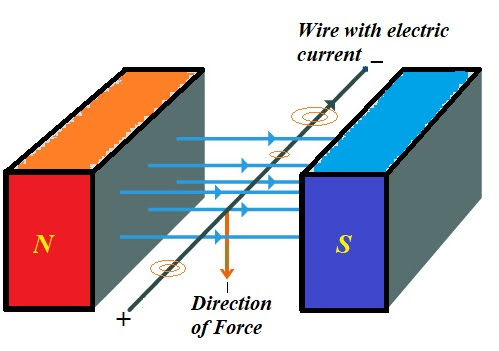The direction of the force can be determined by the "rule of the right
hand", which states that, if the magnetic lines of the external magnetic
field are perpendicularly entering the right hand, while the thumb is
directed towards the electric current in the wire, fingers will show the
direction of force.

A different formulation of the "right hand rule" that results in the
same configuration states that, if magnetic field lines of the external
magnetic field are positioned along the fingers in the direction pointed
by them and the electric current in the wire is running in the
direction of the thumb, then the force exerted by the magnetic field on
the wire is perpendicular to the hand going outside of it.

When the wire has a rectangular shape, as on the picture in the
beginning of this lecture, two side of a rectangle are perpendicular to
the magnetic lines of an external magnetic field. The current in these
wire segments is running in opposite directions. As a result, the force
of magnetic field pushes these sides of a wire in opposite directions,
and the wire will turn until these two opposite forces balance each
other.

The force of an external magnetic field exerted on the wire with electric current running through it is called Lorentz force.

All the above considerations on interaction between an external magnetic field and an electric current are of qualitative character.

Let's address quantitative character of this interaction.

For starter, we will reduce our interest only to a case of a uniform
magnetic field and an infinitesimally thin straight wire running
perpendicularly to the magnetic field lines, like on the picture above.

It is reasonable to assume that the force exerted by a magnetic field acts on each moving electron within a wire.

Considering the force does not exist, if there is no electric current in
a wire (electrons are not moving), but can be observed only when there
is an electric current in a wire, another reasonable assumption is that
the force depends on the speed of moving electrons, which can be
measured as amperage of the electric current.

Experiment shows that the force is proportional to an amperage,
which can be intuitively explained by the idea that the higher the
amperage - the greater "number" of magnetic field lines of an external
magnetic field, crossed by electrons per unit of time, and each such
crossing results in certain incremental increase in the force exerted by
a field.

One more natural assumption is that the longer the wire - the
proportionally greater is the force exerted on it by a magnetic field.
This also is related to the above mentioned idea of a magnetic field
exerting a force on each electron crossing its magnetic field lines.

As a result, we come to a conclusion that the force is proportional to a product of electric current and the length of a wire:

F = b·I·L

where

b is a coefficient of proportionality that characterizes the strength of an external magnetic field,

I is the amperage of an electric current running through a wire,

L is the length of a wire.

units of measurement of the strength of a magnetic field in terms of
units of measurement of force (F), electric current (I) and length (L).

DEFINITION

A uniform magnetic field that exerts a strength of (1N) on a wire of

1 newton1 meter (1m) length with a current running through it perpendicularly to the magnetic lines of a field of 1 ampere (1A) has a strength of 1 tesla (1T).

Tesla is a unit of measurement of the strength of a magnetic field.

The strength of a magnetic field is denoted by a symbol B. The Lorentz force is, therefore, expressed as

F = I·L·B

where

I is the amperage of an electric current running through a wire,

L is the length of a wire,

B is the strength of an external magnetic field

All the above considerations are valid for a case of an electric current
running perpendicularly to lines of a uniform magnetic field.

As mentioned above, the Lorentz force exerted on a wire depends on the movement of electrons in the wire crossing the magnetic lines of an external magnetic field.

Simple geometry prompts us to conclude that, if the direction of the
current is not perpendicular to magnetic lines of an external magnetic
field, but at angle φ with them, the number of magnetic
lines crossed by electrons in a unit of time is smaller and, actually,
is smaller by a factor sin(φ).

So, for any angle φ between the electric current and magnetic field lines of an external magnetic field the formula for Lorentz force would be

F = I·L·B·sin(φ)

where

I is the amperage of an electric current running through a wire,

L is the length of a wire,

B is the strength of an external magnetic field

φ is the angle between the direction of the electric current and lines of an external magnetic field

Taking into consideration the direction of the Lorentz force
perpendicular to both vectors - electric current (from plus to minus)
and lines of an external uniform magnetic field (from South to North),
the above formula can be represented using a vector product

F = I ·L⨯ B